Select Page

# Differential Equations CBSE Maths 12 Science MCQ Answers in English

Differential Equations CBSE Maths 12 Science MCQ Answers in English to enable students to get Answers in a narrative video format for the specific question.

Expert Teacher provides Differential Equations CBSE Maths 12 Science MCQ Answers through Video Answers in English language. This video solution will be useful for students to understand how to write an answer in exam in order to score more marks. This teacher uses a narrative style for a question from Differential Equations not only to explain the proper method of answering question, but deriving right answer too.

Please find the question below and view the Answer in a narrative video format.

Question:

## Similar Questions from CBSE, 12th Science, Maths, Differential Equations

Question 1 : Find the particular solution of the differential equation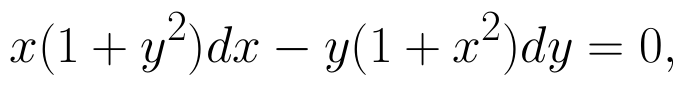given that y = 1 when x = 0.  (View Answer Video)

Question 2 : Find the particular solution of the differential equation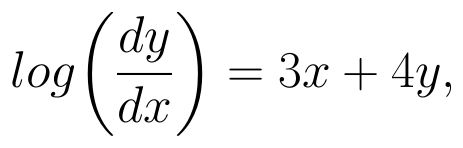given that y = 0, when x = 0. (View Answer Video)

Question 3 : Write the degree of the differential equation :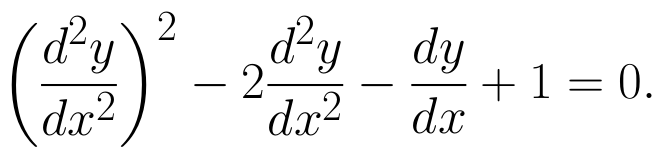(View Answer Video)

Question 4 : Find the sum of the order and the degree of the following differential equation: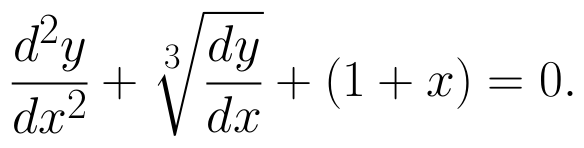(View Answer Video)

### Vector Algebra

Question 1 : Find the unit vector in the direction of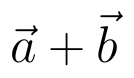if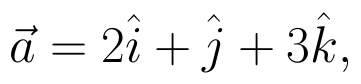and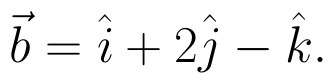(View Answer Video)

Question 2 : Find the magnitude of two vectors a and b having the same magnitude and such that the angle between them is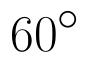and their scalar product is 1/2. (View Answer Video)

Question 3 : Compute the magnitude of the following vectors: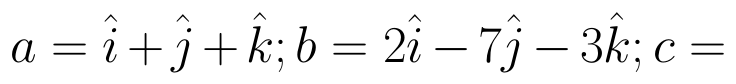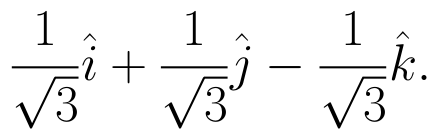(View Answer Video)

Question 4 :  Write a unit vector in the direction of the sum of vectors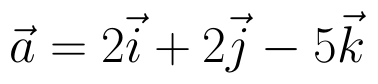and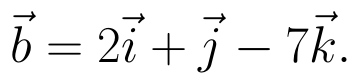(View Answer Video)

Question 5 : Find the area of the triangle with vertices A(1, 1, 2), B(2, 3, 5) and C(1, 5, 5). (View Answer Video)

### Integrals

Question 1 : Find: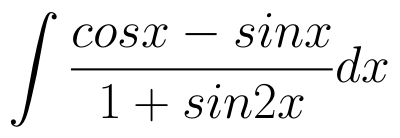. (View Answer Video)

Question 2 : Show that :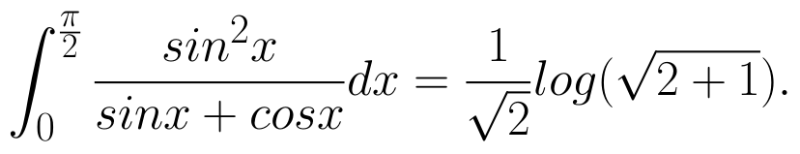(View Answer Video)

Question 3 : Find :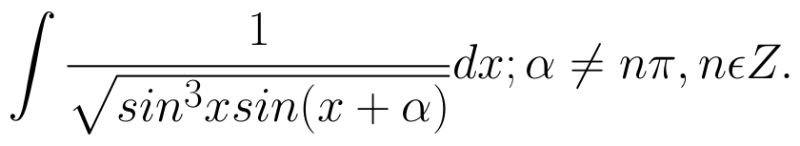(View Answer Video)

Question 4 : Find: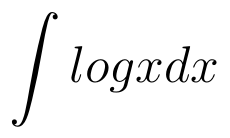. (View Answer Video)

Question 5 : Find the integral of the function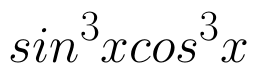. (View Answer Video)

### Probability

Question 1 : Of the students in a college, it is known that 60% reside in hostel and 40% are day scholars (not residing in hostel). Previous year results report that 30% of all students who reside in hostel attain  'A' grade and 20% of day scholars attain 'A' grade in their annual examination. At the end of the year, one student is chosen at random from the college and he has an 'A' grade. What is the probability that the student is a hosteler?   (View Answer Video)

Question 2 : A die is thrown 6 times. If 'getting an odd number' is a 'success', what is the probability of obtaining:
atleast 5 successes?
Question 4 : If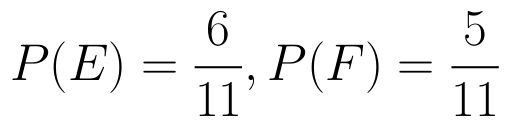and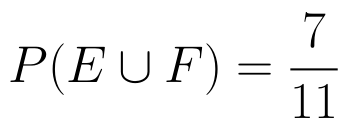then find P(E / F). (View Answer Video)